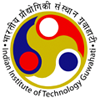•Department of Electronics and Electrical Engineering
Indian Institute of Technology GuwahatiDepartment of Electronics
and Electrical Engineering

### Linear Algebra and Optimization

Course Contents:

Linear Algebra - vector spaces, linear independence, bases and dimension, linear maps and matrices, eigenvalues, invariant subspaces, inner products, norms, orthonormal bases, spectral theorem, isometries, polar and singular value decomposition, operators on real and complex vector spaces, characteristic polynomial, minimal polynomial; optimization - sequences and limits, derivative matrix, level sets and gradients, Taylor series; unconstrained optimization - necessary and sufficient conditions for optima, convex sets, convex functions, optima of convex functions, steepest descent, Newton and quasi Newton methods, conjugate direction methods; constrained optimization - linear and non-linear constraints, equality and inequality constraints, optimality conditions, constrained convex optimization, projected gradient methods, penalty methods.

Texts / References:

1. S. Axler, Linear Algebra Done Right, 2nd Edn., Springer, 1997.
2. E. K. P. Chong and S. H. Zak, An Introduction to Optimization, 2nd Edn., Wiley India Pvt. Ltd., 2010.
3. G. Strang, Linear Algebra and Its Applications, Nelson Engineering, 2007.
4. D. C. Lay, Linear Algebra and Its Applications, 3rd Edition, Pearson, 2002.
5. D. G. Luenberger and Y. Ye, Linear and Nonlinear Programming, 3rd Edn., Springer, 2010.